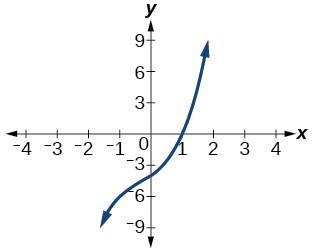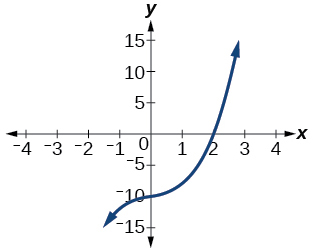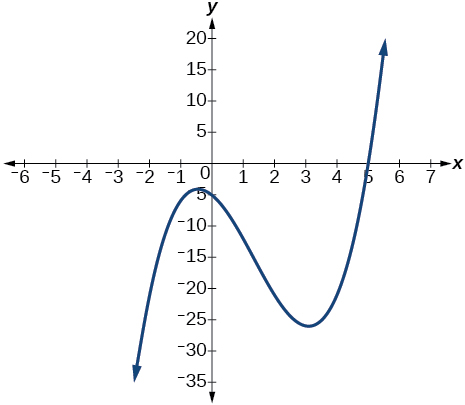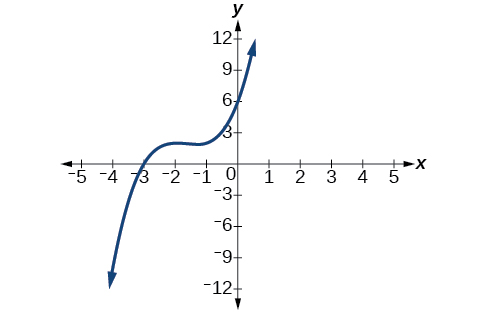# 5.4 Dividing polynomials  (Page 4/6)

 Page 4 / 6

## Verbal

If division of a polynomial by a binomial results in a remainder of zero, what can be conclude?

The binomial is a factor of the polynomial.

If a polynomial of degree $\text{\hspace{0.17em}}n\text{\hspace{0.17em}}$ is divided by a binomial of degree 1, what is the degree of the quotient?

## Algebraic

For the following exercises, use long division to divide. Specify the quotient and the remainder.

$\left({x}^{2}+5x-1\right)÷\left(x-1\right)$

$x+6+\frac{5}{x-1}\text{,}\text{\hspace{0.17em}}\text{quotient:}\text{\hspace{0.17em}}x+6\text{,}\text{\hspace{0.17em}}\text{remainder:}\text{\hspace{0.17em}}\text{5}$

$\left(2{x}^{2}-9x-5\right)÷\left(x-5\right)$

$\left(3{x}^{2}+23x+14\right)÷\left(x+7\right)$

$\left(4{x}^{2}-10x+6\right)÷\left(4x+2\right)$

$\left(6{x}^{2}-25x-25\right)÷\left(6x+5\right)$

$x-5\text{,}\text{\hspace{0.17em}}\text{quotient:}\text{\hspace{0.17em}}x-5\text{,}\text{\hspace{0.17em}}\text{remainder:}\text{\hspace{0.17em}}\text{0}$

$\left(-{x}^{2}-1\right)÷\left(x+1\right)$

$\left(2{x}^{2}-3x+2\right)÷\left(x+2\right)$

$2x-7+\frac{16}{x+2}\text{,}\text{\hspace{0.17em}}\text{quotient:}\text{​}\text{\hspace{0.17em}}2x-7\text{,}\text{\hspace{0.17em}}\text{remainder:}\text{\hspace{0.17em}}\text{16}$

$\left({x}^{3}-126\right)÷\left(x-5\right)$

$\left(3{x}^{2}-5x+4\right)÷\left(3x+1\right)$

$x-2+\frac{6}{3x+1}\text{,}\text{\hspace{0.17em}}\text{quotient:}\text{\hspace{0.17em}}x-2\text{,}\text{\hspace{0.17em}}\text{remainder:}\text{\hspace{0.17em}}\text{6}$

$\left({x}^{3}-3{x}^{2}+5x-6\right)÷\left(x-2\right)$

$\left(2{x}^{3}+3{x}^{2}-4x+15\right)÷\left(x+3\right)$

$2{x}^{2}-3x+5\text{,}\text{\hspace{0.17em}}\text{quotient:}\text{\hspace{0.17em}}2{x}^{2}-3x+5\text{,}\text{\hspace{0.17em}}\text{remainder:}\text{\hspace{0.17em}}\text{0}$

For the following exercises, use synthetic division to find the quotient.

$\left(3{x}^{3}-2{x}^{2}+x-4\right)÷\left(x+3\right)$

$\left(2{x}^{3}-6{x}^{2}-7x+6\right)÷\left(x-4\right)$

$2{x}^{2}+2x+1+\frac{10}{x-4}$

$\left(6{x}^{3}-10{x}^{2}-7x-15\right)÷\left(x+1\right)$

$\left(4{x}^{3}-12{x}^{2}-5x-1\right)÷\left(2x+1\right)$

$2{x}^{2}-7x+1-\frac{2}{2x+1}$

$\left(9{x}^{3}-9{x}^{2}+18x+5\right)÷\left(3x-1\right)$

$\left(3{x}^{3}-2{x}^{2}+x-4\right)÷\left(x+3\right)$

$3{x}^{2}-11x+34-\frac{106}{x+3}$

$\left(-6{x}^{3}+{x}^{2}-4\right)÷\left(2x-3\right)$

$\left(2{x}^{3}+7{x}^{2}-13x-3\right)÷\left(2x-3\right)$

${x}^{2}+5x+1$

$\left(3{x}^{3}-5{x}^{2}+2x+3\right)÷\left(x+2\right)$

$\left(4{x}^{3}-5{x}^{2}+13\right)÷\left(x+4\right)$

$4{x}^{2}-21x+84-\frac{323}{x+4}$

$\left({x}^{3}-3x+2\right)÷\left(x+2\right)$

$\left({x}^{3}-21{x}^{2}+147x-343\right)÷\left(x-7\right)$

${x}^{2}-14x+49$

$\left({x}^{3}-15{x}^{2}+75x-125\right)÷\left(x-5\right)$

$\left(9{x}^{3}-x+2\right)÷\left(3x-1\right)$

$3{x}^{2}+x+\frac{2}{3x-1}$

$\left(6{x}^{3}-{x}^{2}+5x+2\right)÷\left(3x+1\right)$

$\left({x}^{4}+{x}^{3}-3{x}^{2}-2x+1\right)÷\left(x+1\right)$

${x}^{3}-3x+1$

$\left({x}^{4}-3{x}^{2}+1\right)÷\left(x-1\right)$

$\left({x}^{4}+2{x}^{3}-3{x}^{2}+2x+6\right)÷\left(x+3\right)$

${x}^{3}-{x}^{2}+2$

$\left({x}^{4}-10{x}^{3}+37{x}^{2}-60x+36\right)÷\left(x-2\right)$

$\left({x}^{4}-8{x}^{3}+24{x}^{2}-32x+16\right)÷\left(x-2\right)$

${x}^{3}-6{x}^{2}+12x-8$

$\left({x}^{4}+5{x}^{3}-3{x}^{2}-13x+10\right)÷\left(x+5\right)$

$\left({x}^{4}-12{x}^{3}+54{x}^{2}-108x+81\right)÷\left(x-3\right)$

${x}^{3}-9{x}^{2}+27x-27$

$\left(4{x}^{4}-2{x}^{3}-4x+2\right)÷\left(2x-1\right)$

$\left(4{x}^{4}+2{x}^{3}-4{x}^{2}+2x+2\right)÷\left(2x+1\right)$

$2{x}^{3}-2x+2$

For the following exercises, use synthetic division to determine whether the first expression is a factor of the second. If it is, indicate the factorization.

$x-2,\text{\hspace{0.17em}}4{x}^{3}-3{x}^{2}-8x+4$

$x-2,\text{\hspace{0.17em}}3{x}^{4}-6{x}^{3}-5x+10$

Yes $\text{\hspace{0.17em}}\left(x-2\right)\left(3{x}^{3}-5\right)$

$x+3,\text{\hspace{0.17em}}-4{x}^{3}+5{x}^{2}+8$

$x-2,\text{\hspace{0.17em}}4{x}^{4}-15{x}^{2}-4$

Yes $\text{\hspace{0.17em}}\left(x-2\right)\left(4{x}^{3}+8{x}^{2}+x+2\right)$

$x-\frac{1}{2},\text{\hspace{0.17em}}2{x}^{4}-{x}^{3}+2x-1$

$x+\frac{1}{3},\text{\hspace{0.17em}}3{x}^{4}+{x}^{3}-3x+1$

No

## Graphical

For the following exercises, use the graph of the third-degree polynomial and one factor to write the factored form of the polynomial suggested by the graph. The leading coefficient is one.

Factor is $\text{\hspace{0.17em}}{x}^{2}-x+3$Factor is $\text{\hspace{0.17em}}\left({x}^{2}+2x+4\right)$$\left(x-1\right)\left({x}^{2}+2x+4\right)$

Factor is $\text{\hspace{0.17em}}{x}^{2}+2x+5$Factor is $\text{\hspace{0.17em}}{x}^{2}+x+1$$\left(x-5\right)\left({x}^{2}+x+1\right)$

Factor is ${x}^{2}+2x+2$For the following exercises, use synthetic division to find the quotient and remainder.

$\frac{4{x}^{3}-33}{x-2}$

$\text{Quotient:}\text{\hspace{0.17em}}4{x}^{2}+8x+16\text{,}\text{\hspace{0.17em}}\text{remainder:}\text{\hspace{0.17em}}-1$

$\frac{2{x}^{3}+25}{x+3}$

$\frac{3{x}^{3}+2x-5}{x-1}$

$\text{Quotient:}\text{\hspace{0.17em}}3{x}^{2}+3x+5\text{,}\text{\hspace{0.17em}}\text{remainder:}\text{\hspace{0.17em}}0$

$\frac{-4{x}^{3}-{x}^{2}-12}{x+4}$

$\frac{{x}^{4}-22}{x+2}$

$\text{Quotient:}\text{\hspace{0.17em}}{x}^{3}-2{x}^{2}+4x-8\text{,}\text{\hspace{0.17em}}\text{remainder:}\text{\hspace{0.17em}}-6$

## Technology

For the following exercises, use a calculator with CAS to answer the questions.

Consider $\text{\hspace{0.17em}}\frac{{x}^{k}-1}{x-1}\text{\hspace{0.17em}}$ with What do you expect the result to be if $\text{\hspace{0.17em}}k=4?$

Consider $\text{\hspace{0.17em}}\frac{{x}^{k}+1}{x+1}\text{\hspace{0.17em}}$ for What do you expect the result to be if $\text{\hspace{0.17em}}k=7?$

${x}^{6}-{x}^{5}+{x}^{4}-{x}^{3}+{x}^{2}-x+1$

Consider $\text{\hspace{0.17em}}\frac{{x}^{4}-{k}^{4}}{x-k}\text{\hspace{0.17em}}$ for What do you expect the result to be if $\text{\hspace{0.17em}}k=4?$

Consider $\text{\hspace{0.17em}}\frac{{x}^{k}}{x+1}\text{\hspace{0.17em}}$ with What do you expect the result to be if $\text{\hspace{0.17em}}k=4?$

${x}^{3}-{x}^{2}+x-1+\frac{1}{x+1}$

Consider $\text{\hspace{0.17em}}\frac{{x}^{k}}{x-1}\text{\hspace{0.17em}}$ with What do you expect the result to be if $\text{\hspace{0.17em}}k=4?$

## Extensions

For the following exercises, use synthetic division to determine the quotient involving a complex number.

$\frac{x+1}{x-i}$

$1+\frac{1+i}{x-i}$

$\frac{{x}^{2}+1}{x-i}$

$\frac{x+1}{x+i}$

$1+\frac{1-i}{x+i}$

$\frac{{x}^{2}+1}{x+i}$

$\frac{{x}^{3}+1}{x-i}$

${x}^{2}-ix-1+\frac{1-i}{x-i}$

## Real-world applications

For the following exercises, use the given length and area of a rectangle to express the width algebraically.

Length is $\text{\hspace{0.17em}}x+5,\text{\hspace{0.17em}}$ area is $\text{\hspace{0.17em}}2{x}^{2}+9x-5.$

Length is area is $\text{\hspace{0.17em}}4{x}^{3}+10{x}^{2}+6x+15$

$2{x}^{2}+3$

Length is $\text{\hspace{0.17em}}3x–4,\text{\hspace{0.17em}}$ area is $\text{\hspace{0.17em}}6{x}^{4}-8{x}^{3}+9{x}^{2}-9x-4$

For the following exercises, use the given volume of a box and its length and width to express the height of the box algebraically.

Volume is $\text{\hspace{0.17em}}12{x}^{3}+20{x}^{2}-21x-36,\text{\hspace{0.17em}}$ length is $\text{\hspace{0.17em}}2x+3,\text{\hspace{0.17em}}$ width is $\text{\hspace{0.17em}}3x-4.$

$2x+3$

Volume is $\text{\hspace{0.17em}}18{x}^{3}-21{x}^{2}-40x+48,\text{\hspace{0.17em}}$ length is $\text{\hspace{0.17em}}3x–4,\text{\hspace{0.17em}}$ width is $\text{\hspace{0.17em}}3x–4.$

Volume is $\text{\hspace{0.17em}}10{x}^{3}+27{x}^{2}+2x-24,\text{\hspace{0.17em}}$ length is $\text{\hspace{0.17em}}5x–4,\text{\hspace{0.17em}}$ width is $\text{\hspace{0.17em}}2x+3.$

$x+2$

Volume is $\text{\hspace{0.17em}}10{x}^{3}+30{x}^{2}-8x-24,\text{\hspace{0.17em}}$ length is $\text{\hspace{0.17em}}2,\text{\hspace{0.17em}}$ width is $\text{\hspace{0.17em}}x+3.$

For the following exercises, use the given volume and radius of a cylinder to express the height of the cylinder algebraically.

Volume is $\text{\hspace{0.17em}}\pi \left(25{x}^{3}-65{x}^{2}-29x-3\right),\text{\hspace{0.17em}}$ radius is $\text{\hspace{0.17em}}5x+1.$

$x-3$

Volume is $\text{\hspace{0.17em}}\pi \left(4{x}^{3}+12{x}^{2}-15x-50\right),\text{\hspace{0.17em}}$ radius is $\text{\hspace{0.17em}}2x+5.$

Volume is $\text{\hspace{0.17em}}\pi \left(3{x}^{4}+24{x}^{3}+46{x}^{2}-16x-32\right),\text{\hspace{0.17em}}$ radius is $\text{\hspace{0.17em}}x+4.$

$3{x}^{2}-2$

f(x)=x/x+2 given g(x)=1+2x/1-x show that gf(x)=1+2x/3
proof
AUSTINE
sebd me some questions about anything ill solve for yall
how to solve x²=2x+8 factorization?
x=2x+8 x-2x=2x+8-2x x-2x=8 -x=8 -x/-1=8/-1 x=-8 prove: if x=-8 -8=2(-8)+8 -8=-16+8 -8=-8 (PROVEN)
Manifoldee
x=2x+8
Manifoldee
×=2x-8 minus both sides by 2x
Manifoldee
so, x-2x=2x+8-2x
Manifoldee
then cancel out 2x and -2x, cuz 2x-2x is obviously zero
Manifoldee
so it would be like this: x-2x=8
Manifoldee
then we all know that beside the variable is a number (1): (1)x-2x=8
Manifoldee
so we will going to minus that 1-2=-1
Manifoldee
so it would be -x=8
Manifoldee
so next step is to cancel out negative number beside x so we get positive x
Manifoldee
so by doing it you need to divide both side by -1 so it would be like this: (-1x/-1)=(8/-1)
Manifoldee
so -1/-1=1
Manifoldee
so x=-8
Manifoldee
Manifoldee
so we should prove it
Manifoldee
x=2x+8 x-2x=8 -x=8 x=-8 by mantu from India
mantu
lol i just saw its x²
Manifoldee
x²=2x-8 x²-2x=8 -x²=8 x²=-8 square root(x²)=square root(-8) x=sq. root(-8)
Manifoldee
I mean x²=2x+8 by factorization method
Kristof
I think x=-2 or x=4
Kristof
x= 2x+8 ×=8-2x - 2x + x = 8 - x = 8 both sides divided - 1 -×/-1 = 8/-1 × = - 8 //// from somalia
Mohamed
hii
Amit
how are you
Dorbor
well
Biswajit
can u tell me concepts
Gaurav
Find the possible value of 8.5 using moivre's theorem
which of these functions is not uniformly cintinuous on (0, 1)? sinx
which of these functions is not uniformly continuous on 0,1
solve this equation by completing the square 3x-4x-7=0
X=7
Muustapha
=7
mantu
x=7
mantu
3x-4x-7=0 -x=7 x=-7
Kr
x=-7
mantu
9x-16x-49=0 -7x=49 -x=7 x=7
mantu
what's the formula
Modress
-x=7
Modress
new member
siame
what is trigonometry
deals with circles, angles, and triangles. Usually in the form of Soh cah toa or sine, cosine, and tangent
Thomas
solve for me this equational y=2-x
what are you solving for
Alex
solve x
Rubben
you would move everything to the other side leaving x by itself. subtract 2 and divide -1.
Nikki
then I got x=-2
Rubben
it will b -y+2=x
Alex
goodness. I'm sorry. I will let Alex take the wheel.
Nikki
ouky thanks braa
Rubben
I think he drive me safe
Rubben
how to get 8 trigonometric function of tanA=0.5, given SinA=5/13? Can you help me?m
More example of algebra and trigo
What is Indices
If one side only of a triangle is given is it possible to solve for the unkown two sides?
cool
Rubben
kya
Khushnama
please I need help in maths
Okey tell me, what's your problem is?
NavinByByByByBy